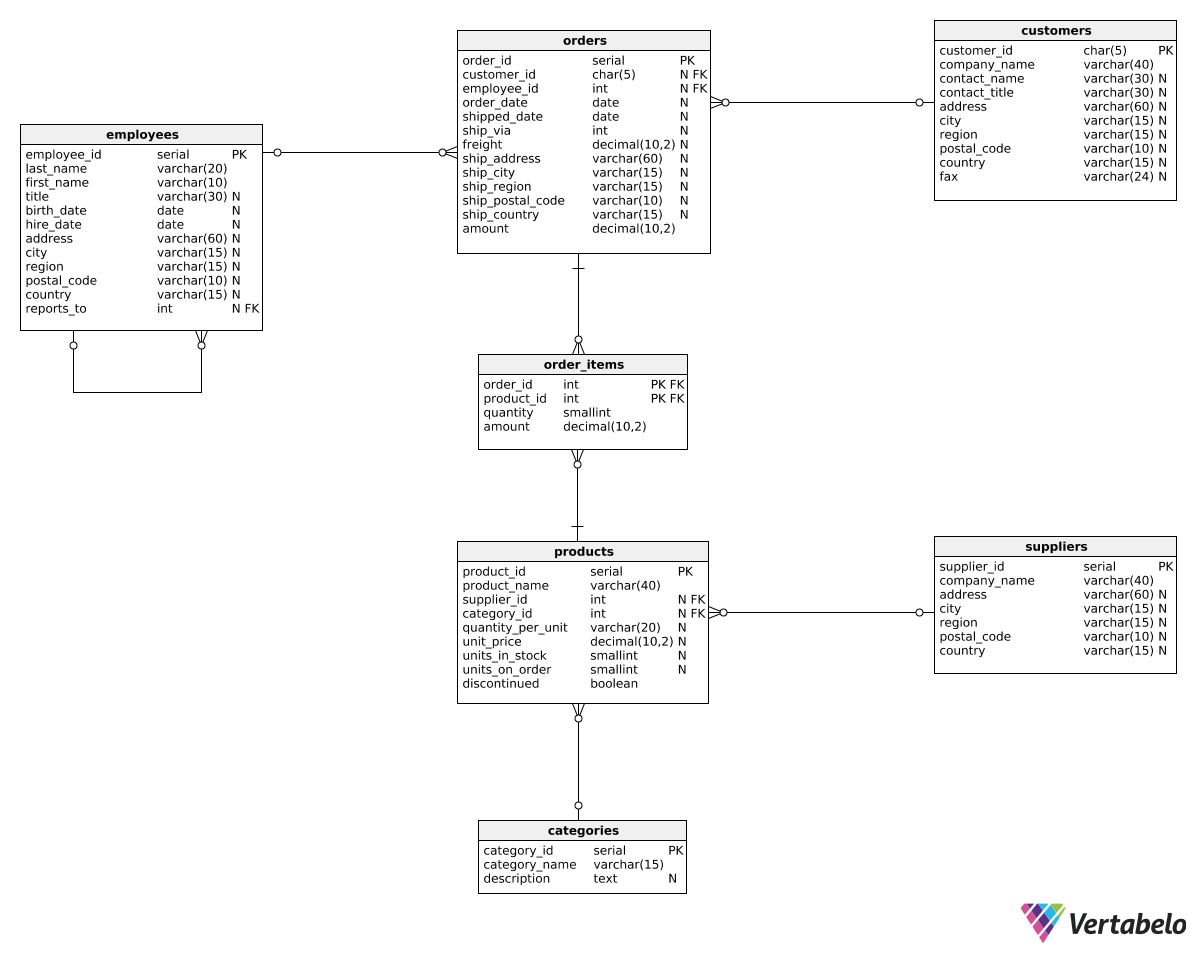Deals Of The Week - hours only!Up to 80% off on all courses and bundles.-Close
Introduction
Total revenue for multiple periods
Calculating deltas
Creating "revenue in quarters" reports
Summary

## Instruction

Very well done! Let's try one more exercise before we move on.

## Exercise

For each month of 2017, calculate the total monthly revenue from orders shipped to the USA and the percentage revenue change (delta) as compared to the previous month. Show three columns: revenue_month, total_revenue, and delta_percentage.

In the first row, leave the delta value as NULL. Order the rows by month. Here's how you can count delta_percentage:

\frac{\textrm{total amount} - \textrm{total amount from preceding months}}{\textrm{total amount from preceding months}} \cdot 100

Remember to round the result to the second decimal point.

### Stuck? Here's a hint!

Use the following expression in the last column:

ROUND(
100 *
(SUM(amount) - LAG(SUM(amount), 1) OVER (ORDER BY EXTRACT(month FROM order_date)))
/ CAST(LAG(SUM(amount), 1) OVER (ORDER BY EXTRACT(month FROM order_date)) AS decimal(10, 2)),
2) AS delta_percentage